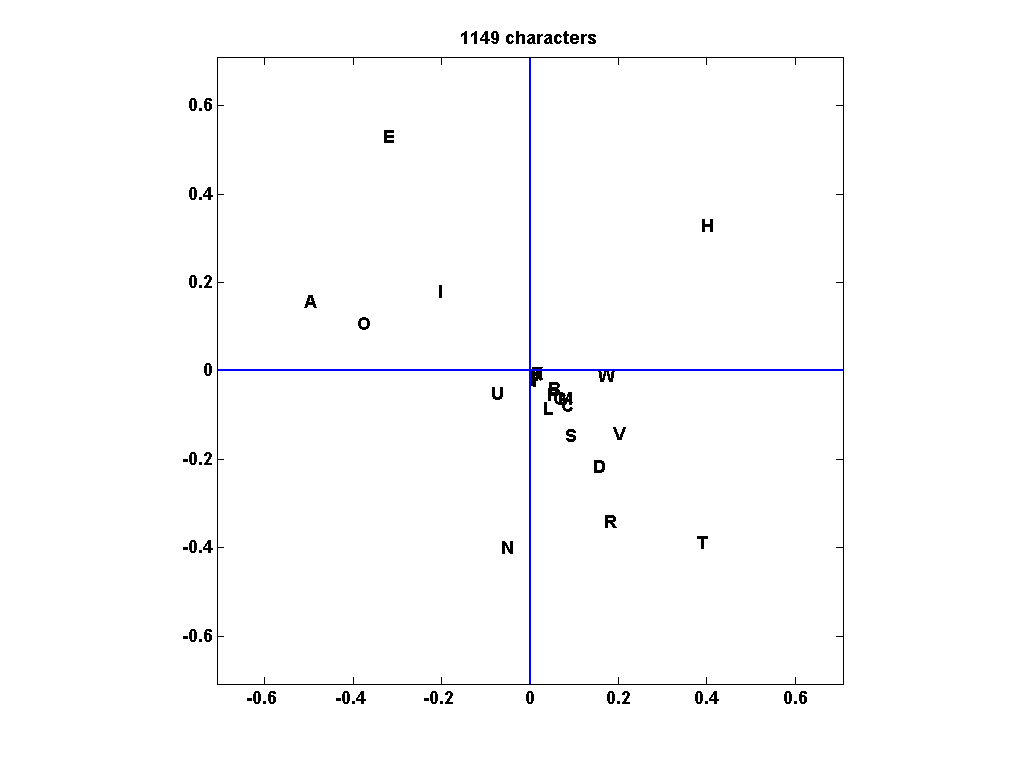# Singular Value Analysis of Cryptograms

The Singular Value Decomposition of the digram frequency matrix of a text coded with a simple substitution cypher can reveal information about the vowels and consonants in the text.

### Contents

#### Don Morrison

I have mentioned Don Morrison before in Cleve's Corner, in my post on Pythagorean Addition. He was the mathematician and computer scientist who recruited me to the University of New Mexico in 1972. He was remarkably imaginative and today's post is about another one of his creative ideas. We wrote a paper about it, cited below, in the American Math Monthly thirty years ago.

#### Digram frequency matrix

In English, and in many other languages, vowels are usually followed by consonants and consonants are usually followed by vowels. This fact is reflected by the principal component analysis of what I will call the digram frequency matrix for a sample of text. (I am using the term digram to refer to any pair of letters, although in the discipline of orthography the term more precisely refers to a pair of characters that represents one phoneme.) English text uses 26 letters, so the digram frequency matrix is a 26-by-26 matrix, $A$, with counts of pairs of letters. Blanks and all other punctuation are removed from the text and the entire sample is thought of as circular or periodic, so the first letter follows the last letter. The matrix entry $a_{i,j}$ is the number of times the $i$-th letter is followed by the $j$-th letter. The row and column sums of $A$ are the same; they count the number of times individual letters occur in the sample. The fifth row and fifth column usually have the largest sums because the fifth letter, which is "E," is usually the most frequent.

#### Principal component analysis

A principal component analysis of $A$ produces a first component, $$A \approx \sigma_1 u_1 v_1^T$$ that reflects the individual letter frequencies. The first right- and left-singular vectors, $u_1$ and $v_1$, have elements that are all of the same sign and that are roughly proportional to the corresponding frequencies. We are primarily interested in the second principal component, $$A \approx \sigma_1 u_1 v_1^T + \sigma_2 u_2 v_2^T$$ The second term has positive entries in vowel-consonant and consonant-vowel positions and negative entries in vowel-vowel and consonant-consonant positions. The sign patterns adjust the frequency counts to reflect the vowel-consonant properties of the language.

My Numerical Computing with MATLAB toolbox ncm includes a function, digraph.m, that carries out this analysis. Let's run the function on Lincoln's Gettysburg Address, gettysburg.txt.
   fid = fopen('gettysburg.txt');
fclose(fid);

Convert to integers between 1 and 26.
   k = upper(char(txt)) - 'A' + 1;
k(k < 1 | k > 26) = [];

Use the counting feature of sparse to generate the digram frequency matrix.
   j = k([2:length(k) 1]);
A = full(sparse(k,j,1,26,26));
cnt = sum(A);

Compute the SVD.
   [U,S,V] = svd(A);


#### Plot

Plot the second left and right singular vectors. The $i$-th letter of the alphabet is plotted at coordinates $(u_{i,2},v_{i,2})$. The distance of each letter from the origin is roughly proportional to its frequency, and the sign patterns cause the vowels to be plotted in one quadrant and the consonants to be plotted in the opposite quadrant. There is more detail. The letter "N" is usually preceded by a vowel and often followed by another consonant, like "D" or "G," and so it shows up in a quadrant pretty much by itself, sort of a "left vowel, right consonant" . On the other hand, "H" is often preceded by another consonant, namely "T," and followed by a vowel, "E," so it also gets its own quadrant, a "right vowel, left consonant".
   for i = 1:26
if cnt(i) > 0
text(U(i,2),V(i,2),char('A'+i-1))
end
end

s = 4/3*max(max(abs(U(:,2))),max(abs(V(:,2))));
axis(s*[-1 1 -1 1])
axis square
line([0 0],[-s s],'color','b')
line([-s s],[0 0],'color','b')
box
title(sprintf('%d characters',length(k)))#### Cryptograms

A simple substitution cipher is obtained by permuting the letters of the alphabet. The digram matrix $A$ is replaced by $PAP^T$ where $P$ is a permutation matrix. The singular vectors are simply multiplied by $P$. Letters in the plot are permuted, but the locations and quadrants remain the same. So it is possible to identify the vowels and consonants, and perhaps -- if the sample is large enough -- the letters standing for "N" and "H" in the cryptogram. I admit that simple substitution ciphers are not the world's most difficult codes to crack, but I found this to be an unexpected use of the SVD.

#### Reference

Cleve Moler and Donald Morrison, "Singular Value Analysis of Cryptograms", The American Mathematical Monthly, Vol. 90, No. 2, 1983, pp. 78-87, <http://www.jstor.org/stable/2975804>

Published with MATLAB® R2014a
|